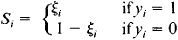# random number generator

(redirected from Random generator)
Also found in: Dictionary, Thesaurus, Medical.

## random number generator

[′ran·dəm ′nəm·bər ‚jen·ə‚rād·ər]
(computer science)
A mathematical program which generates a set of numbers which pass a randomness test.
An analog device that generates a randomly fluctuating variable, and usually operates from an electrical noise source.

## Random Number Generator

a device for the generation of random numbers that are uniformly distributed over a given range of numbers. Its uses include the simulation of the actual operating conditions of automatic control systems, the solution of problems by the Monte Carlo method, and the modeling of random changes of production parameters in automated control systems. Besides their direct use in statistical models, the uniformly distributed random numbers produced by a random number generator can be used to form number sequences having a specified distribution law.

The principal unit in a random number generator is a generator of equally probable random digits, from which the required multidigit combinations of digits, or numbers, are formed. Most generators produce binary digits. Various primary sources of random signals are used in random digit generators—for example, the intrinsic noise of special resistors or of electron-tube, gas-discharge, or semi-conductor devices. Other sources of random signals include α –, β–, and γ-radioactivity and fluctuations in the phase and amplitude of harmonic oscillations. The random digit generator contains an appropriate device for shaping the initial signals, which is called the source of the primary stochastic process. The generator also includes the following: a shaping amplifier, which converts the initial stochastic process into a form that is convenient for digital interpretation; an analog-to-digital converter of the shaped random signals into discrete equally probable states of some electronic device (such as a flip-flop), where each state corresponds to a certain digit; and a probability stabilizer, which ensures the stability of the probability characteristics of the generated sequence of digits.

One common stabilization method is based on a combination of direct and inverted representations of the generated digits. In this case, the stabilized sequence S1, S2, …, Si … is formed from the basic sequence ξ12 … ξi, … and the control sequence y1, y2, …, yi, … according to the ruleDepending on the method of forming the multidigit random numbers from the elementary sequences of equally probably digits, random number generators are divided into sequential and parallel types; a composite type making use of both methods is also distinguished. The sequential type has only one random digit generator. In this case, an n-position random number (that is, a number with n digits) is formed by filling in turn each position of the corresponding register. In the parallel type, each digit of the number being formed has its own generator, and all the digits are entered simultaneously in the register. The parallel type generates random numbers more quickly but requires more complicated equipment than does the sequential type. This disadvantage, however, may not be important when integrated circuits are used.

### REFERENCES

Bobnev, M. P. Generirovanie sluchainykh signalov, 2nd ed. Moscow, 1971.
Iakovlev, V. V., and R. F. Fedorov. Stokhasticheskie vychislitel’nye mashiny. Leningrad, 1974.

I. A. DANIL’CHENKO

## random number generator

A software routine that produces a random number. Used in applications such as computer games and cryptographic key generation, random numbers are easily created in a computer due to many random events that take place. For example, only the difference of a few milliseconds between keystrokes is enough to seed a random number generation routine with a different starting number each time.

Once seeded, an algorithm computes different numbers throughout the session. The numbers that are created must be distributed evenly over a certain range, and they cannot be predictable (the next number cannot be determined from the last).
References in periodicals archive ?
The use of prerecorded random numbers obviates the need for a true random generator, because the required random numbers can be obtained from the Internet.
In addition to these precautions, the following randomness check was specifically tailored to the situation in which the random generator was to be used.
The random generator (similar to the one in Schmidt, 1970) used the random timing of radioactive decays (average of 30 signals per second from an Sr-90 source registered by a Geiger counter) to stop a fast counter (0.
The evaluation makes no assumptions about the reliability of the experimenter's random generator.
The true random generator utilized the timing of radioactive decay as the basic source of randomness (Schmidt, 1970) to generate binary random bit-sequences.
After the experimenter sent the sealed printout to the home address of one independent observer (Rudolph), the other independent observer (Morris) used his own random generator to print out the sequence of target assignments.
The true random generator was provided by a noise source that activated a flip-flop at random time intervals, at an average rate of about 1,000 flips/second.
In our setting, perfect randomness of the true random generator was not a critical matter.

Site: Follow: Share:
Open / Close